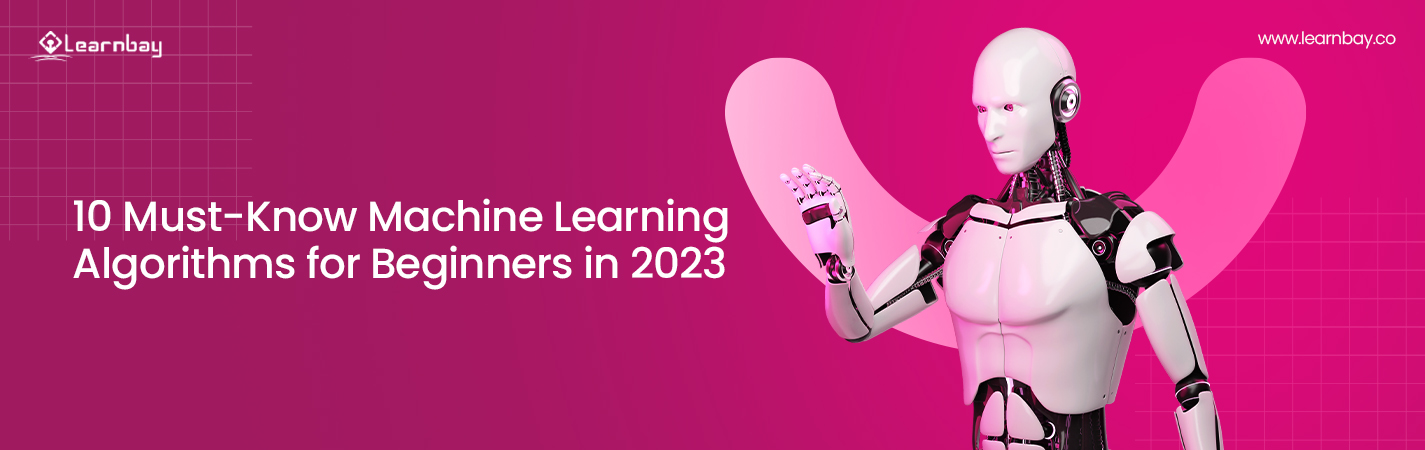# 10 Must-Know Machine Learning Algorithms for Beginners in 2023

By Nivin Biswas Published in Machine Learning 15-18 mins

Know the 10 Most Useful Machine Learning Algorithms That Every Beginner Should Include in Their Learning List

The idea of manual labor has completely altered, and society is moving toward an automated world. Numerous ML techniques have boosted computing intelligence and adaptability.

With the advancement of machine learning technology and the spread of its benefits to various domains, the demands of machine learning engineers are touching the sky. In such a scenario opting for a machine learning career transition seems an intelligent decision.

But as a beginner, it's not a good idea to get confused between tons of machine learning algorithms.

Here, we'll take a closer look at the 10 most useful machine learning algorithms that have been developed internationally to tackle more challenging real-world issues. These are the basic ML algorithm. Once you master these 10 gems, you can quickly adapt to any other machine learning algorithms, as those are nothing but the modification of these 10.

## The 10 Elementary But Most Useful Machine Learning Algorithms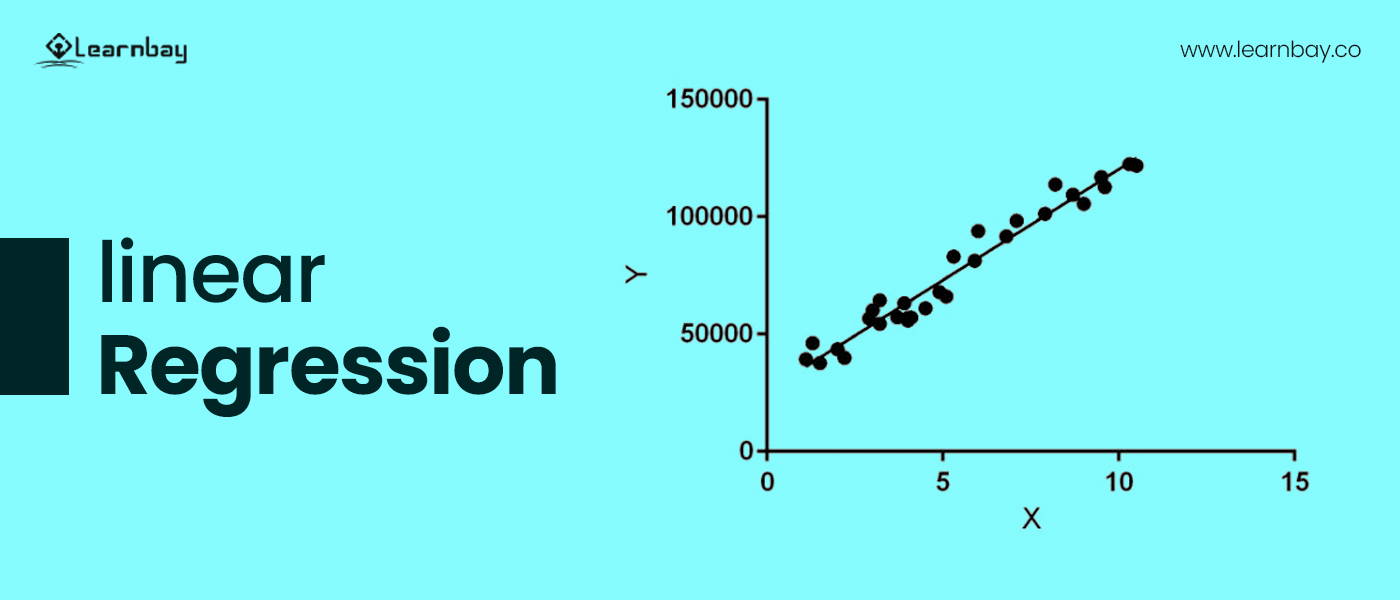### 1. Linear regression:-

One of the simplest and most straightforward machine learning algorithms for predicting continuous variables. Such an ML algorithm starts with learning from independent variables. This is called linear regression in Machine learning. Additionally, a dependent variable is an effect whose value changes along with the independent variable.

The linear equation concerning the relationship between dependent and independent variables is:

• Y=a*X+b represents this regression line.

• X is defined as an Independent variable

• Y is defined as a dependent variable

• a is defined as a Slope

• b is defined as an Intercept

Example:

Suppose an ed-tech platform launched new courses a few months back. The course is going well. But the company is not sure if they should raise the course fee now or should wait for a few more months.

If the course sales have been increasing steadily over the past few months, a linear regression analysis of the sales data and monthly sales can be used to forecast future sales.

### 2. SVM algorithm

The support vector machine (SVM) is a method of classifying data points by assigning them to specific coordinates on an n-dimensional space, making it easy to balance and focus different datasets and find trends in the data. With certain data sets, the support vector machine can reduce data complexity.

## The SVM algorithm is classified into two types:-

### Linear SVM:-

Linear SVM is mainly used for data that can be divided into two categories using a single straight line. The most common application of this line is to separate two classes. This is performed by employing the Linear SVM classifier.

### Non-Linear SVM:-

The phrase non-linear SVM refers to data that cannot be categorized within a straight line. This is a primary classifier for use with the non-linear SVM classifier.

Example:

We can use SVM algorithms in a variety of ways, but the most common is to classify documents into various categories in a variety of ways.

For example, a news article can be of various types, but if you want to learn about the business journal, it will help you to classify the data set in order to provide you with better results.

### 3. Decision tree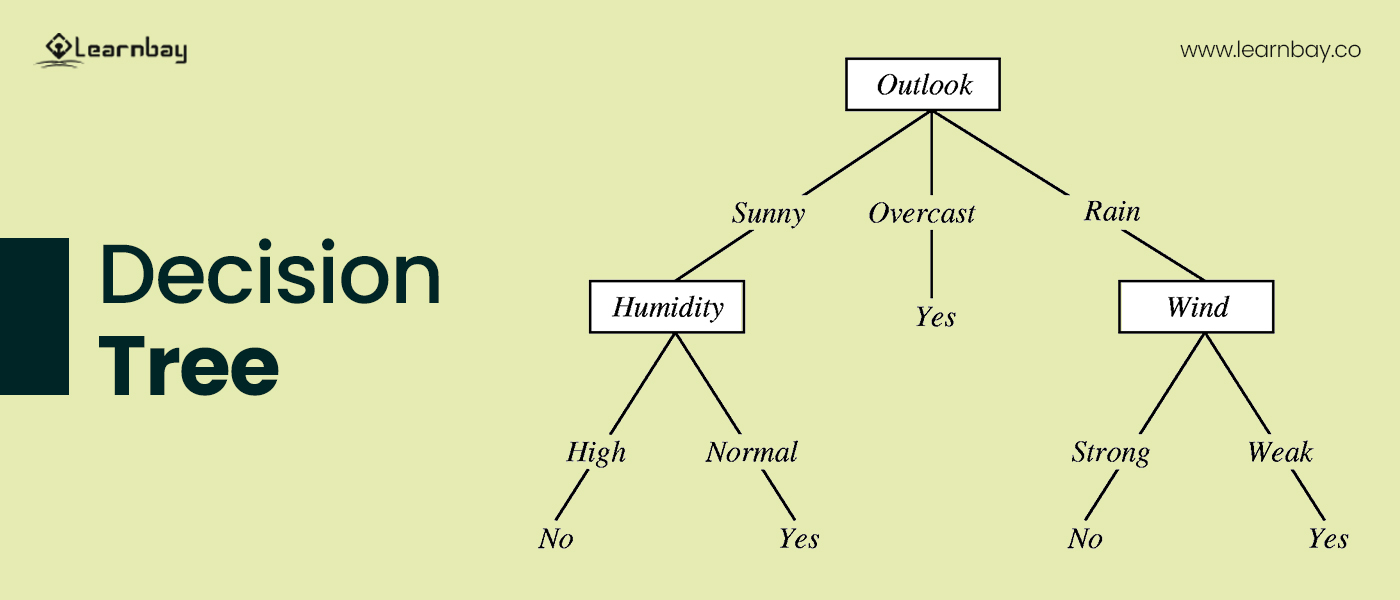A decision tree ML algorithms is a type of predictive modeling that is used to categorize data and forecast future outcomes. This approach works by learning the characteristics of the data and answering the query in order to make better decisions. This algorithm can help with both categorical and continuous dependent variables. The decision tree's methods are listed below.

• Root node(Stating input)

• Leaf node(Final output)

• parent node/ child node

• Splitting

• Pruning

• Sub tree

Example:

If we want to buy any product online, we will get several suggestions on the online product based on previous purchases and searches.

### 4. Logistic regression machine learning algorithm:-

Logistic regression machine learning is a method used to predict discrete values from a set of independent variables using binary values such as 0 and 1. A logistic regression algorithm can easily forecast and estimate the likelihood that an event will occur by fitting the data in the logit function. In each equation, we can easily derive the logistic regression. The following are some of the steps to obtaining logistic regression.

Here we have known fact that the linear regression is written as:-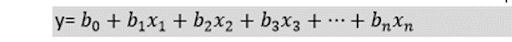And, because Y in logistic regression can range between 0 and 1, we will separate and divide the above equation with (1-y):-intoHowever, in order to obtain the final product, we must first define a range of - [infinity] to +[infinity] and then adjust the equation to obtain the final result.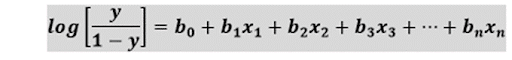And the final equation of logistic regression is defined above.

Example:

Logistic regression can assist in sorting and classifying data. For example, if you receive a variety of emails, logistic regression can assist in classifying the emails and distinguishing which are spam and which are legitimate.

### 5. KNN algorithm:-

KNN stands for the K-Nearest Neighbors algorithm, a statistical method that examines how closely two objects can be placed next to one another. It is a sign that the data points in this algorithm have similarities if they are close to each other. This technique can be used to combine various data points. Below is the working of the K- NN algorithm:-

The working of K-NN is explained below and is relatively straightforward:-

Step 1 - We need to select various sorts of K of the Neighbors

Step 2 - The second step is to calculate the Euclidean distance between K numbers of neighbors.

Step 3 - Next, we need to search for the K closest neighbor based on the calculated Euclidean distance.

Step 4: Here, we need to count the number of data points in each k category.

Step 5 - We need to assess the new data points to categorize the maximum number of k neighbors.

Step 6: Here, we can easily calculate and finalize the model.

Example:

The KNN algorithm aids in the forecasting and prediction of data sets. The KNN algorithm can be used to forecast the weather or predict stock market prices etc.

### 6. K-means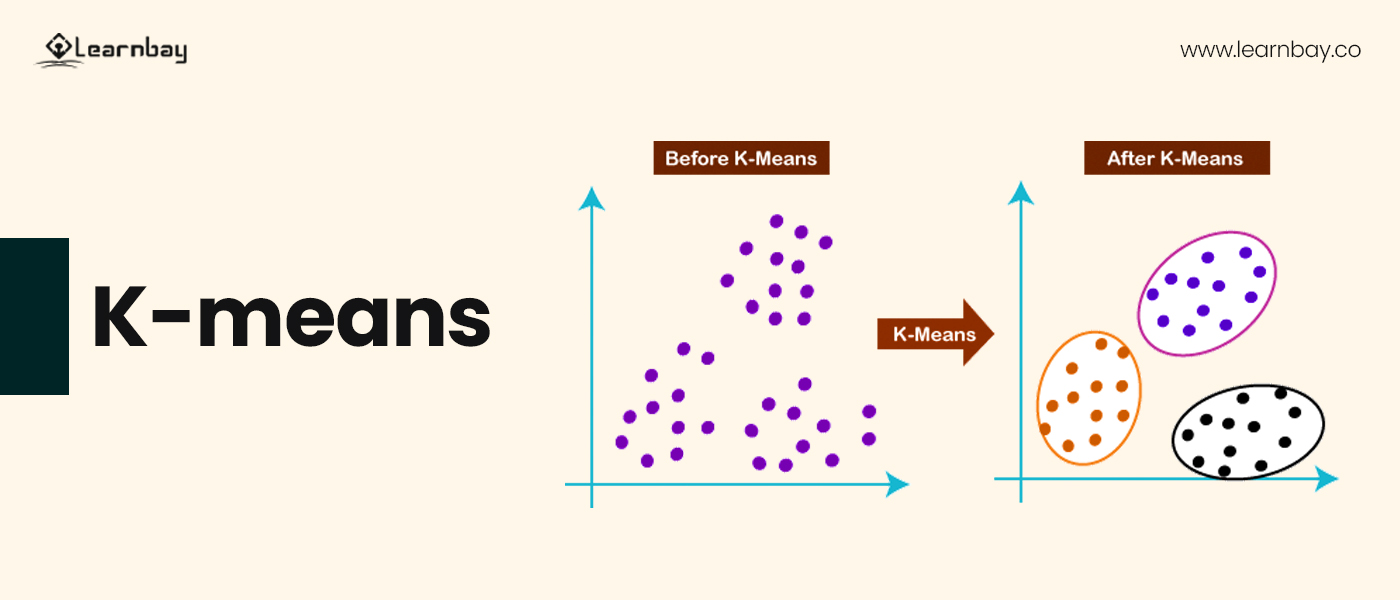A k-means clustering algorithm is an effective tool for data-organized classification. It is easy to use and organize data into a preset number of clusters, ensuring that each cluster contains only homogenous and distinct data points. This project is an innovative approach to solving a clustering problem.

The K means algorithm is demonstrated below and operates in the following ways:

Step 1: We must decide and sort the amount of k and cluster required.

Step 2 - In the second step, we must select the random k points to form the datasets.

Step 3 :- It becomes indispensable to assist the data point to their closest centroid, forming a predefined k cluster.

Step 4 - We need to find and calculate the variance to the centroid in each cluster.

Step 5: In the fifth step, we must repeat the third step, i.e., assigning each data point.

Step 6: If any reallocation or reassignment is needed, we must precisely go to step 4.

Step 7 - We must confirm the model assignment and complete the model after completing the sixth step.

Example:

If you listen to music of the same genre frequently, Spotify will recommend similar music based on a customer segmentation algorithm.

### 7. Naive Bayes algorithm:-

A simple machine learning tool called a Naive Bayes classifier makes predictions by assuming that each function must be independent of the others. And even if the features are related to a particular class, the statisticians will nevertheless consider them independently when calculating the likelihood of a result.

A Naive Bayesian model that can outperform more complex models is straightforward to construct. It is a fantastic and effective option for handling massive data collection.

The Naive Bayesian model works on a simple formula, i.e.,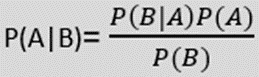Where,

P(A|B) quantifies the probability of hypothesis (A)on the observed event of B.

P(B|A) measures the likelihood that a particular hypothesis is correct based on the available evidence.

P(A) is the measured probability of the hypothesis

P(B) is the measure of observing evidence

Example:

If you are on the field and playing football, but the weather is sunny, you can get a prediction to find out the possible outcome by having a probability that the players should play or not. It works in probabilistic order.

### 8. Random forest algorithm

A group of decision trees is another name for a random forest. The categories and votes assigned to each tree in a random forest are unique to that tree. The Forest then selects the classification that received the most votes after reviewing all of the votes. Below is the working of the Random forest tree.

Step 1: Start with picking a random K at random from the data points.

Step 2: We must now construct the decision tree linked to the chosen data points.

Step 3: In this step, we must use a decision tree and a number (N).

Step 4: Repetition of steps 1 and 2 is required.

Step 5: Assign the new data points to the categories that receive the most support after collecting the new data points, making each decision, assigning the tree's predictions, and determining each decision.

Example:

If you are purchasing a stock, you can easily forecast whether the stock price will rise or fall, allowing us to approximate loss and profit margins.

### 9. Dimensionality reduction algorithms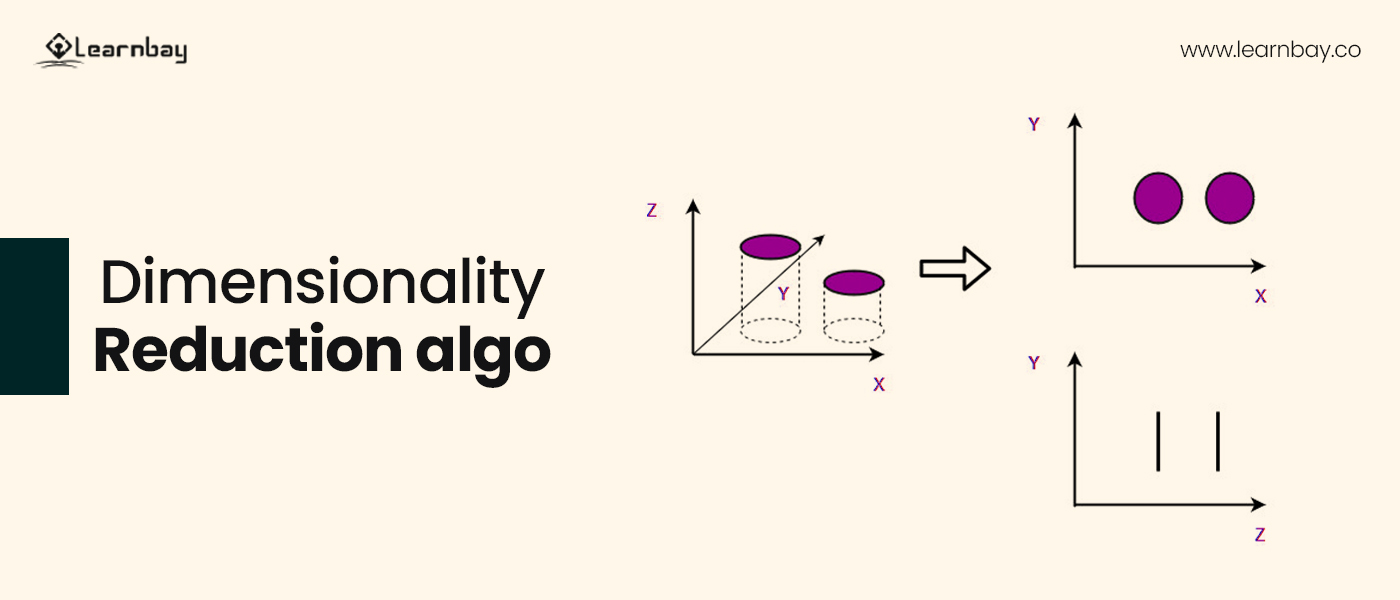A significant amount of data is being gathered across the network every day. Dimensionality reduction algorithms must be started to organize this data and effectively grasp it as a group.

Dimensionality reduction algorithms include Numerous technologies, such as the decision tree approach, the missing value ratio, and the random forest algorithm, which is significantly capable of handling and sorting a large amount of data.

Let's use an example to understand this better.

Example:

If you had a data collection with 500 columns, the density reduction technique would reduce it to 50. However, you must first input a relevant research result or feature from the set of features.

When dealing with massive amounts of data, the gradient boosting algorithm and the AdaBoosting algorithm typically operate effectively and fluidly. It uses a single algorithmic framework to combine decision trees, random forests, Bayesian regression, and multilayer perceptrons.

Example:

If you want to know whether a customer is likely to leave or minimize a company service, you can use the AdaBoost algorithm to solve classification problems by combining R and Python.

## Summing up:-

So there you have it: the machine learning algorithms that every enthusiast in this field should be familiar with. In the machine learning interview for entry-level positions, these 10 ML algorithms remain the focus. So you must hold a good grip on these algorithms. If you want to master these key skills you can opt for advanced AI and ML Certification.